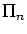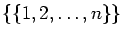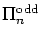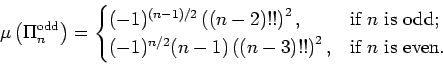Journal of Integer Sequences, Vol. 21 (2018), Article 18.9.6

## An Elementary Proof of the Explicit Formula for the Möbius Number of the Odd Partition Poset

### Kenneth M. Monks Department of Mathematics Front Range Community College -- Boulder County Campus 2190 Miller Drive Longmont, CO 80537 USA

Abstract:

The Möbius number of a finite poset is a very useful combinatorial invariant of the poset that generalizes the classical number-theoretic Möbius function. The Möbius number of the poset of partitionsof a set with n elements is well-known. A related poset, the subposet consisting only of partitions that use odd part size or the maximum element, written, arises in similar combinatorial settings. In this paper, we compute the Möbius numbers of allas follows:This result was first stated as known by Stanley and has since been proven by Sundaram and Wachs. They constructed versions of the formula above by respectively using symmetric function/representation theory and topological/simplicial complex techniques. In this paper, we provide a new proof using only elementary combinatorial techniques and the WZ algorithm.

Full version:  pdf,    dvi,    ps,    latex

(Concerned with sequence A000246.)

Received April 18 2018; revised version received October 16 2018. Published in Journal of Integer Sequences, December 13 2018.